# The efficiency of the CORDIC Operator in the MIMO MC-CDMA receiver

The efficiency of the CORDIC Operator in the MIMO MC-CDMA receiver

Youness MehdaouiAbdessamad Malaoui1 Ahmed Gaga Rachid El Alami Mostafa Mrabti

Research team in electronics, instrumentation and measurements, USMS, Béni-mellal 23030, Morocco

Computer and interdisciplinary physics laboratory, USMBA, Fez 30003, Morocco

LESSI Laboratory, Faculty of Sciences Dhar El Mehraz, Sidi Mohamed Ben Abdellah University, Fez 30003, Morocco

Corresponding Author Email:
youness.mehdaoui@gmail.com
Page:
99-104
|
DOI:
https://doi.org/10.18280/mmep.060113
24 July 2018
|
Accepted:
26 February 2019
|
Published:
31 March 2019
| Citation

OPEN ACCESS

Abstract:

In recent years, there is an increased requirement including transfers rapidity of information, due to the widespread use of multimedia applications on wireless communication system. The MIMO technology offers a promising solution for increasing spectral efficiency and transmitting data across a multi-antenna transmission and reception network. The goal of this study is to improve the data processing time, which poses a big problem of digital communication. In this article, a solution is proposed to improve this problem. The adopted approach shows that the use of the CORDIC algorithm to calculate the cosine and sine of the DFT / IDFT is very efficient and that the use of the fixed point gives a remarkable speed while maintaining a better accuracy. In addition, the use of a powerful fixed-point processor dedicated to signal processing could also have given better results than other results obtained in the literature. The results obtained conclude that the use of the CORDIC algorithm on a fixed point is faster compared to receiver without the CORDIC algorithm.

Keywords:

MIMO MC-CDMA, CORDIC, DFT, fixed point, processing time

1. Introduction

Wireless digital transmission techniques have undergone a great revolution in recent years; this revolution comes on the one hand from the growing demand for telecommunication and data exchange while benefiting from new services such as television, digital radio, wireless local area networks, broadband Internet, mobile telephony and many other multimedia applications. The designers of wireless transmission systems seek to optimize the quality of service and overcome the problems encountered in the design and implementation of their systems.

The digital wireless revolution is emerging in the emergence of several new technologies that significantly increase transmission rates and improve the quality of transmission. Among these technologies, we selected MIMO (Multiple Input Multiple Output) technology and Multi-Carrier Code Division Multiple Access (MC-CDMA) technology for our study.

MIMO technology offers a promising solution for increasing spectral efficiency and transmitting data across a multi-antenna transmission and reception network. Indeed, it offers high throughput and good transmission quality thanks to spatial diversity. However, an important source of performance degradation in MIMO systems is the frequency selectivity of the channel. OFDM-based techniques are promising solutions to combat this selectivity. Indeed, the OFDM modulation transforms a frequency-selective channel into several nonselective sub-channels into frequencies.

The MC-CDMA technique is the combination of the CDMA access technique and the OFDM modulation where the data spread is performed in the frequency domain. The CDMA access technique allows multiple users to share the same radio channel at the same time and at the same frequency while ensuring the separation of their data through the use of orthogonal spreading codes.

The objective of this work is to study and implement a wireless system using the MIMO technique associated with the MC-CDMA technique in order to combine the advantages of these two techniques in a single system called MIMO MC- CDMA.

This paper is organized as follows: In section 2, we explain the MIMO MC-CDMA theory and the CORDIC algorithm. The fix-point development is given in section 3. The proposed MIMO MC-CDMA receiver and the results obtained by the implementation of this receiver on C64x+ are presented in section 4, 5 and 6 respectively. We finish this paper by a conclusion (section 7).

2. Theory

2.1 Design of the MIMO chain associated with MC-CDMA

A MIMO system has several transmit antennas and several antennas at the reception. The two main advantages of MIMO systems are to offer spatial diversity on transmission and reception; it consists of receiving several replicas of the emitted signal affected by independent fading. The order of diversity is equal to the number of independent channels on receipt [1, 2].

The idea of associating the spatial multiplexing technique with the MC-CDMA technique consists first of all in spreading the data of Nu user by their spreading sequences. The symbols obtained are subsequently demultiplexed on the M transmit antennas. On each antenna, the data undergo OFDM modulation.

At reception, the processing of the received symbols is carried out in a first step by the OFDM demodulation on each receiving antenna, then, the symbols obtained are detected by equalization techniques, the symbols are then reordered by a spatial decoding and despread.

The structure of the MIMO MC-CDMA chain is presented in the figures 1 and 2.

## 1.png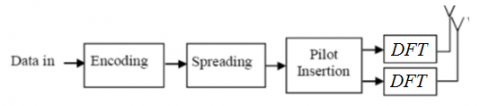Figure 1. Simple model of MIMO MC-CDMA transmitter

## 2.png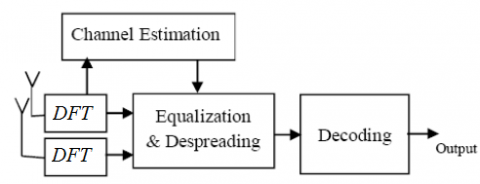Figure 2. Simple model of MIMO MC-CDMA receiver

The transmitted signal after modulation can be expressed as Eq. (1).

$s\left( t \right)=\sum\limits_{i=-\infty }^{\infty }{\sqrt{\frac{2{{E}_{b}}}{{{N}_{c}}{{T}_{s}}}}}\sum\limits_{k=1}^{{{N}_{T}}}{\sum\limits_{n=1}^{{{N}_{C}}}{{{b}_{k}}(i){{c}_{n}}{{\mu }_{{{T}_{S}}}}(t-i{{T}_{s}})\cos ({{\omega }_{n}}t)}}$ (1)

where:

${{N}_{c}}$: number of MC-CDMA system subcarrier

${{N}_{T}}x{{N}_{R}}$: consider the MIMO system

${{E}_{b}}$ : the bit energy

${{T}_{s}}$ : symbol duration

${{\mu }_{{{T}_{S}}}}(t)$: a rectangular waveform with amplitude 1

${{T}_{s}}$: pulse duration

${{b}_{k}}(i)$ The ith transmitted data bit

${{c}_{n}}$: The spreading code

${{N}_{T}}$: The transmitting antenna

${{\omega }_{n}}=2\pi {{f}_{0}}+2\pi (n-1)\Delta f$: The radian frequency of the nth subcarrier

$\Delta f={}^{1}/{}_{{{T}_{s}}}$: The frequency spacing

$r(t)$: The received signal through receiving antenna

${{N}_{R}}$: number of receiver antenna

The received signal can be written in the following form Eq. (2):

$r\left( t \right)=\eta (t)+\sum\limits_{i=-\infty }^{\infty }{\sqrt{\frac{2{{E}_{b}}}{{{N}_{c}}{{T}_{s}}}}}\sum\limits_{k=1}^{{{N}_{T}}}{\sum\limits_{n=1}^{{{N}_{C}}}{{{h}_{n}}{{b}_{k}}(i){{c}_{n}}{{\mu }_{{{T}_{S}}}}(t-i{{T}_{s}})\cos ({{\omega }_{n}}t+{{\varphi }_{n}})}}$(2)

where

${{h}_{n}}$ The subcarrier flat fading gain

${{\varphi }_{n}}$ The subcarrier-fading phase

$\eta (t)$ is AWGN channel

${{N}_{0}}$ Single-sided power spectral density

After phase compensation, amplitude correction is performed by the receiver using the equalization coefficient.

After the application of the DFT the received signal is given by Eq. (3).

$Y(k)=X(k)H(k)+W(k),k=0,1,....,{{N}_{n}}-1$  (3)

The extraction of Y(k) gives the received pilot signal Yp(k), with the knowledge of the channel responses H(k), obtaining the channel transfer function H(k) results from the information carried by Hp(k), The transmitted data samples signal X(k) can be recovered by simply dividing the received signal by channel response  as Eq. (4).

$X(k)=\frac{Y(k)}{H(k)}$ (4)

The equalizer co-efficient is expresses as Eq. (5):

${{\alpha }^{'}}=R_{yy}^{-1}{{R}_{by}}$ (5)

Rby is the constructive cross correlation matrix that contains the ρuk elements of Ryy.

Ryy is the cross correlation matrix of modulated signature waveform.

2.2 CORDIC algorithm

As explained in ; The CORDIC algorithm provides an iterative method of performing vector rotations by arbitrary angles using only shifts and adds . Eq. (6) and Eq. (7) show the basic equations required to implement CORDIC as in :

\begin{align} & \text{X}\left( \text{i}+\text{1} \right)=\text{X}\left( \text{i} \right)\cos \varphi -Y\left( i \right)\sin \varphi \\ & \text{Y}\left( \text{i}+\text{1} \right)=\text{Y}\left( \text{i} \right)\cos \varphi +X\left( i \right)\sin \varphi \\\end{align} (6)

\begin{align} & \text{X}\left( \text{i}+\text{1} \right)=\cos \varphi (X\left( i \right)-Y\left( i \right)\tan \varphi ) \\ & \text{Y}\left( \text{i}+\text{1} \right)=\cos \varphi (Y\left( i \right)+X\left( i \right)\tan \varphi ) \\\end{align}   (7)

If the rotation angles are restricted so that $\tan \varphi =\pm {{2}^{e-1}}$ the multiplication by the tangent term is reduced to simple shift operation , we can be written as in Eq(8) and Eq(9):

\begin{align} & \text{X}\left( \text{i}+\text{1} \right)={{K}_{i}}(X\left( i \right)-Y\left( i \right).{{d}_{i}}{{.2}^{e-i}}) \\ & \text{Y}\left( \text{i}+\text{1} \right)={{K}_{i}}(Y\left( i \right)-X\left( i \right){{d}_{i}}{{.2}^{e-i}}) \\\end{align} (8)

$\text{Z}\left( \text{i}+\text{1} \right)=Z\left( i \right)-{{d}_{i}}.\varphi$ (9)

where

${{K}_{i}}=\cos ({{\tan }^{-1}}({{2}^{e-1}}))$

We can ignore Ki in the iterative process, it can be noted that ${{K}_{i}}=\text{0}\text{.6073}$and ${{d}_{i}}=-1$ if $Z\left( i \right)<0$ , $\pm 1$ otherwise.

which finally provides the following result (Eq. (10) and Eq. (11)):

\begin{align} & {{\text{X}}_{\text{n}}}={{A}_{n}}\left[ {{X}_{0}}\cos {{Z}_{0}}-{{Y}_{0}}.\sin {{Z}_{0}} \right] \\ & {{\text{Y}}_{\text{n}}}={{A}_{n}}\left[ {{Y}_{0}}\cos {{Z}_{0}}+{{X}_{0}}.\sin {{Z}_{0}} \right] \\ & {{Z}_{\text{n}}}=0 \\\end{align} (10)

${{A}_{n}}=\prod\limits_{n}{{}}\sqrt{\text{(1+}{{\text{2}}^{\text{2i}}}\text{)}}$ (11)

So to reach an expected angle, a series of iterations are required to be performed and in this design the number of iterations are $i=8$ and in every iteration the new values of x, y and z depend upon the previous values of the same .

2.3 The calculus of cosine and sinus

It is shown that the rotational mode CORDIC operation can simultaneously compute the sine and cosine of the input angle as in  by Eq. (12):

\begin{align} & {{\text{X}}_{\text{n}}}={{A}_{n}}.{{X}_{0}}\cos {{Z}_{0}} \\ & {{\text{Y}}_{\text{n}}}={{A}_{n}}.{{X}_{0}}\sin {{Z}_{0}} \\\end{align} (12)

By setting ${{X}_{\text{0}}}=1/{{A}_{n}}$, the rotation produces the un-scaled sine and cosine of the angle argument${{Z}_{o}}$ . It is worth noting that the hardware complexity of the CORDIC rotator is approximately equivalent to that of a single multiplier with the same word size .

3. The Development of Fixed Point

The diagram below illustrates a typical development scenario in use today

## 3.png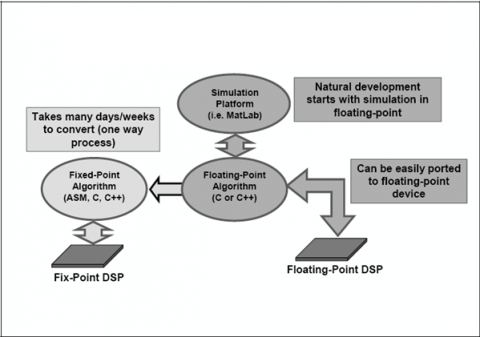Figure 3. The dilemma of fixed point development

The design may initially start with a simulation (i.e. MatLab) of a control algorithm, which typically would be written in floating-point math (C or C++). Existing methodologies [8, 9] achieve a ﬂoating-to-ﬁxed-point transformation leading to an ANSI-C code with integer data types. This algorithm can be easily ported to a floating-point device. However, because of the commercial reality of cost constraints, most likely a 16-bit or 32-bit fixed-point device would be used in many target systems.

The effort and skill involved in converting a floating-point algorithm to function using a 16-bit or 32-bit fixed-point device is quite significant. A great deal of time (many days or weeks) would be needed for reformatting, scaling and coding the problem. Additionally, the final implementation typically has little resemblance to the original algorithm .

For digital signal processors (DSPs), the methodology aim is to deﬁne the optimized ﬁxed point speciﬁcation which minimizes the code execution time and leads to sufficient accuracy , some experiments  can represent up to 30% of the global implementation time.

4. Proposed Software-Hardware Implementation

Future wireless communication systems need improvement in spectral efficiency (increased of rate of flow), the following diagram shows the MIMO MC-CDMA receiver using CORDIC fixed point in order to increase the processing rates.

In this work, we are interested to implement a MIMO MC-CDMA receiver using a DSP device.

The following diagram shows MIMO MC-CDMA receiver using CORDIC algorithm and fixed point.

## 4.pngFigure 4. The Architecture of MIMO MC-CDMA Receiver using CORDIC into fixed point

In our systems based on the MIMO MC-CDMA method, every sequence is encoded by 16 chips Walsh-Hadamard sequence like , and each column of emission matrix is modulated by two IDFT. We propose a MIMO MC-CDMA receiver based on CORDIC algorithm and Fixed Point using a DSP (TMS320C64x+).

Table 1. Parameters

 Channel Rayleigh fading Modulation BPSK/16 QAM/64QAM Antennas 2x2 Equalization/Estimation MMSE/Pilot DFT size 512 Spreading Codes Walsh-Hadamard Code
5. Implementation

5.1 Hardware implementation

The algorithms are implemented using DSP Processor, the DSP based on 65-nm process technology and 3.0 GHz of total raw DSP processing power with performance of up to 24,000 million instructions per second (MIPS) [or 24,000 16-bit MMACs per cycle , the C6474 device offers cost-effective solutions to high-performance DSP programming challenges with three independent DSP subsystems. The DSP possesses the operational flexibility of high-speed controllers and numerical capability of array processors .

The eight functional units (.M1, .L1, .D1, .S1, .M2, .L2, .D2, and .S2) are each capable of executing one instruction every clock cycle. The .M functional units perform all multiply operations . The .S and .L units perform a general set of arithmetic, logical, and branch functions. The .D units primarily load data from memory to the register file and store results from the register file into memory .

## 5.png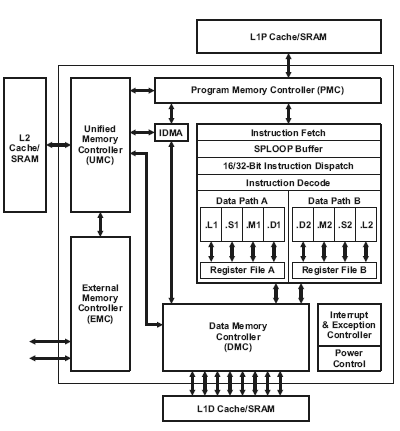Figure 5. TMS320C64x+ DSP Block Diagram

5.2 Software implementation

We used for the simulations Code Composer Studio software  which uses more efficiently the internal hardware of the C64x+. The implementation of the MIMO MC-CDMA receiver based of CORDIC algorithm on a DSP with fixed point. To calculate the sine and cosine values that are needed to calculate the twiddle factors in DFT for MIMO MC-CDMA Receiver we used the CORDIC algorithm.

Architecture for DFT has been presented in . It has been observed that as the number of N-point samples increase, the time and hardware requirements of the system increase. Faster algorithms like Fast Fourier transform (FFT) can solve this problem .

The algorithm for the software implementation of the MIMO MC-CDMA receiver is given below:

a) Remove from the signal received from the channel the cyclic prefix.

i) Remove from the received signal, the first M samples of the (N + M) samples, where N is the actual number of input samples and M is the cyclic prefix length and

b) Equalization of the channel

c) The samples obtained.in step a) will be computed the DFT

i) Figure 6 show the flow diagram for DFT Computation.

In the butterfly calculation part of the flow chart the CORDIC custom instruction is used.

d) Demodulate by DFT a signal obtained in step c) to obtain the spreading signal bits.

## 6a.png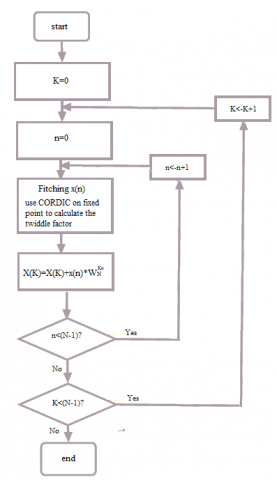Figure 6. Diagram for DFT computation

6. Results

On the DSP C64x+ the MIMO MC-CDMA receiver is implemented and tested for different lengths of input data. For a DFT length of 64 points the following results are obtained; the clock cycle is equal to 1 GHz.

The three figures 7, 8 and 9 show the number of cycles obtained for the three receivers without CORDIC and with CORDIC.

The table 2 show the cycles number and their ratios without CORDIC and with CORDIC. The results are given on fixed point and floating point for the proposed MIMO MC-CDMA receiver, OFDM receiver and RAKE receiver given in .

The results obtained by implementation on DSP C64x+ of the proposed MIMO MC-CDMA receiver are compared to the literature results .

## 7.png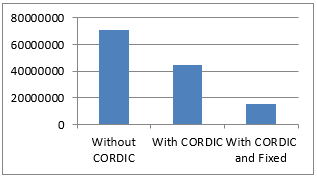Figure 7. The number of cycles obtained for the MIMO MC-CDMA receiver

## 8.png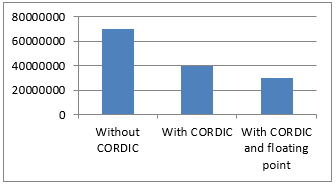Figure 8. The number of cycles obtained for the OFDM receiver

## 9.png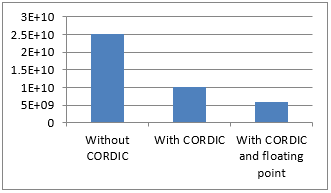Figure 9. The number of cycles obtained for the RAKE receiver

Table 2. The cycle’s number and their ratios

 MIMO MC-CDMA receiver This work OFDM receiver RAKE receiver Benchmark (cycles) Without CORDIC 71203561 70000000 25000000000 With CORDIC 44944552 40000000 10000000000 With CORDIC and Fixed point or floating point 15256849 (fixed point) 30000000 (floating point) 6000000000 (floating point) Ratio Without CORDIC/ With CORDIC and Fixed point or floating point 4.67 1.33 1.66

## 10.png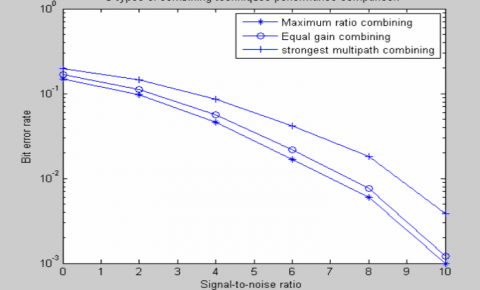Figure 10. SNR vs BER plot for RAKE receiver performance evaluation

## 11.pngFigure 11. SNR vs BER plot for OFDM performance evaluation

## 12.png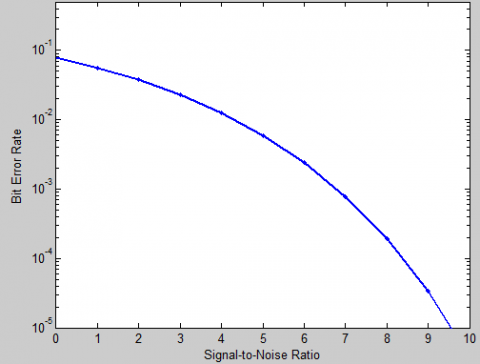Figure 12. SNR vs BER plot for MIMO MC-CDMA performance evaluation

The performance of the MIMO MC-CDMA receiver, OFDM receiver and RAKE receiver  is illustrated using bit error rate (BER) calculations.

The figure 10, 11 and 12 show the bit error rate (BER) against signal to noise ratio (SNR).

It is shown in figures 10, 11 and 12 that the proposed architecture of the MIMO MC-CDMA receiver than the OFDM receiver and RAKE receiver of  and give the same performance obtained by .

The proposed new architecture provides better computational speed by keeping a performance efficiency compared to the results found in .

Also, the cycle number of MIMO MC-CDMA receiver (15256849) represents just 19.66% of the cycle number of OFDM receiver (30000000) and 3.93% of RAKE receiver (6000000000) in .

7. Conclusions

In this work, the approach that has been adopted shows that the use of the CORDIC algorithm to calculate the cosine and sine of the DFT / IDFT is very efficient and the use of the fixed point gives a remarkable speed while keeping a better precision. Also the use of a powerful fixed-point processor dedicated to the signal processing could have had better results than other results obtained in the literature.

According to the obtained results in our implementation, we can conclude that using the CORDIC algorithm on fixed point is faster (with a ratio of 4.67) compared to receiver without CORDIC algorithm.

The perspective work is the implementation of the system in a hybrid circuit containing Field Programmable Gate Arrays (FPGA) and DSP.

References

 Berder O. (2002). Optimisation et stratégie d'allocation de puissance des systèmes de transmission multi-antennes. Thesis at the University of Western Brittany, France.

 Kammoun-Jemal I. (2004). Codage spatio-temporel sans connaissance à priori du canal. Thesis at the National School of Telecommunications of Paris, France.

 Hsieh MH, Wei CH. (1998). Channel estimation for OFDM systems based on comb-type pilot arrangement in frequency selective fading channels". IEEE Transactions on Consumer Electronics 44(1): 217-225. https://doi.org/10.1109/30.663750

 Chaitanya KS, Muralidhar P, Rama Rao CB. (2009). Implementation of CORDIC based RAKE receiver architecture. 2nd IEEE International Conference on Computer Science and Information Technology, Beijing, China. https://doi.org/10.1109/ICCSIT.2009.5234625

 Mehdaoui Y, Mrabti M. (2010). A faster MC-CDMA system using a DSP implementation of the FFT. 5th International Symposium On I/V Communications and Mobile Network, Rabat, Morocco. https://doi.org/10.1109/ISVC.2010.5656245

 Andraka R. (1998). A survey of CORDIC algorithms for FPGA based computers. Proc. of the 1998 CM/SIGDA Sixth International Symposium on FPGAs, Monterey, CA, pp. 191-200. https://doi.org/10.1145/275107.275139

 Srinivasa Chaitanya K, Muralidhar P, Rama Rao CB. (2009). Implementation of Cordic based architecture for WCDMA/OFDM Receiver, European Journal of Scientific Research 36(1): 65-78.

 Kum KI, Kang J, Sung W. (2000). AUTOSCALER for C: an optimizing ﬂoating-point to integer C program converter for ﬁxed-point digital signal processors. IEEE Transactions on CircuitsandSyst—PartII 47(9): 840-848. https://doi.org/10.1109/82.868453

 Willems M, Bursgens V, Meyr H. (1997). FRIDGE ﬂoating point programming of ﬁxed-point digital signal processors. In Proceeding of 8th International Conference on Signal Processing Applications and Technology (ICSPAT ’97), San Diego, Calif, USA.

 DSPArithmeticTutorial (2008). Texas Instrument.

 Menard D, Chillet D, Sentieys O. (2006), Floating-to-fixed-point conversion for digital signal processors. EURASIP Journal on Applied Signal Processing 2006: 1–19. https://doi.org/10.1155/ASP/2006/96421

 Grotker T, Multhaup E, Mauss O. (1996), Evaluation of HW/SW tradeoffs using behavioral synthesis. In Proceeding of 7th International Conference on Signal Processing Applications and Technology (ICSPAT’96), Boston, Mass, USA, pp. 781-785.

 TMS320C6474 Multicore Digital Signal Processor. (2008). Texas Instrument.

 Code Composer Studio v3.3. (2008). Texas Instrument.

 De D, Gaurav Kumar K, Ghosh R, Saha A. (2017). FPGA implementation of discrete fourier transform using CORDIC algorithm. Advances in Modelling and Analysis B 60(2): 332-337. https://doi.org/10.18280/ama_b.600205

 Mehdaoui Y, El Alami R. (2018). DSP implementation of the Discrete Fourier Transform using the CORDIC algorithm on fixed point. Advances in Modelling and Analysis B 61(3): 123-126. https://doi.org/10.18280/ama_b.610303